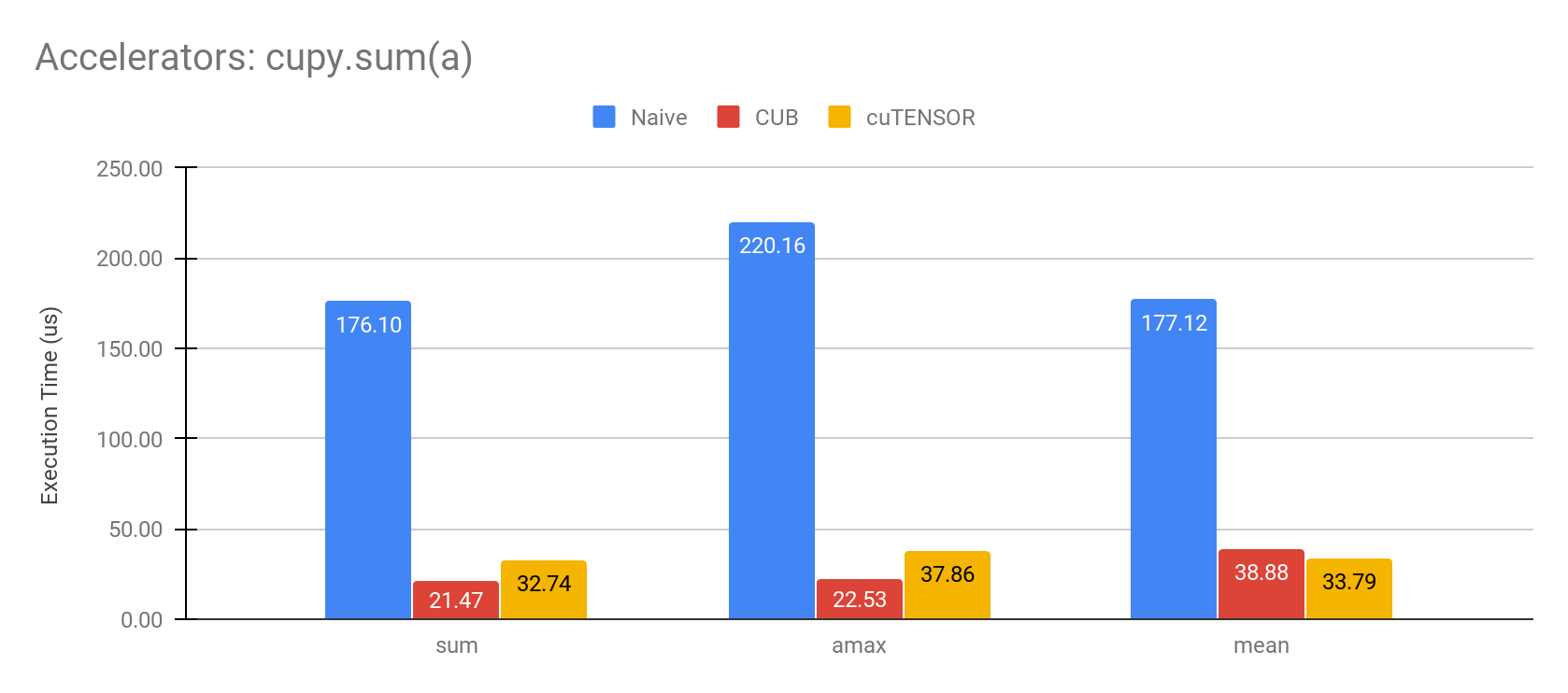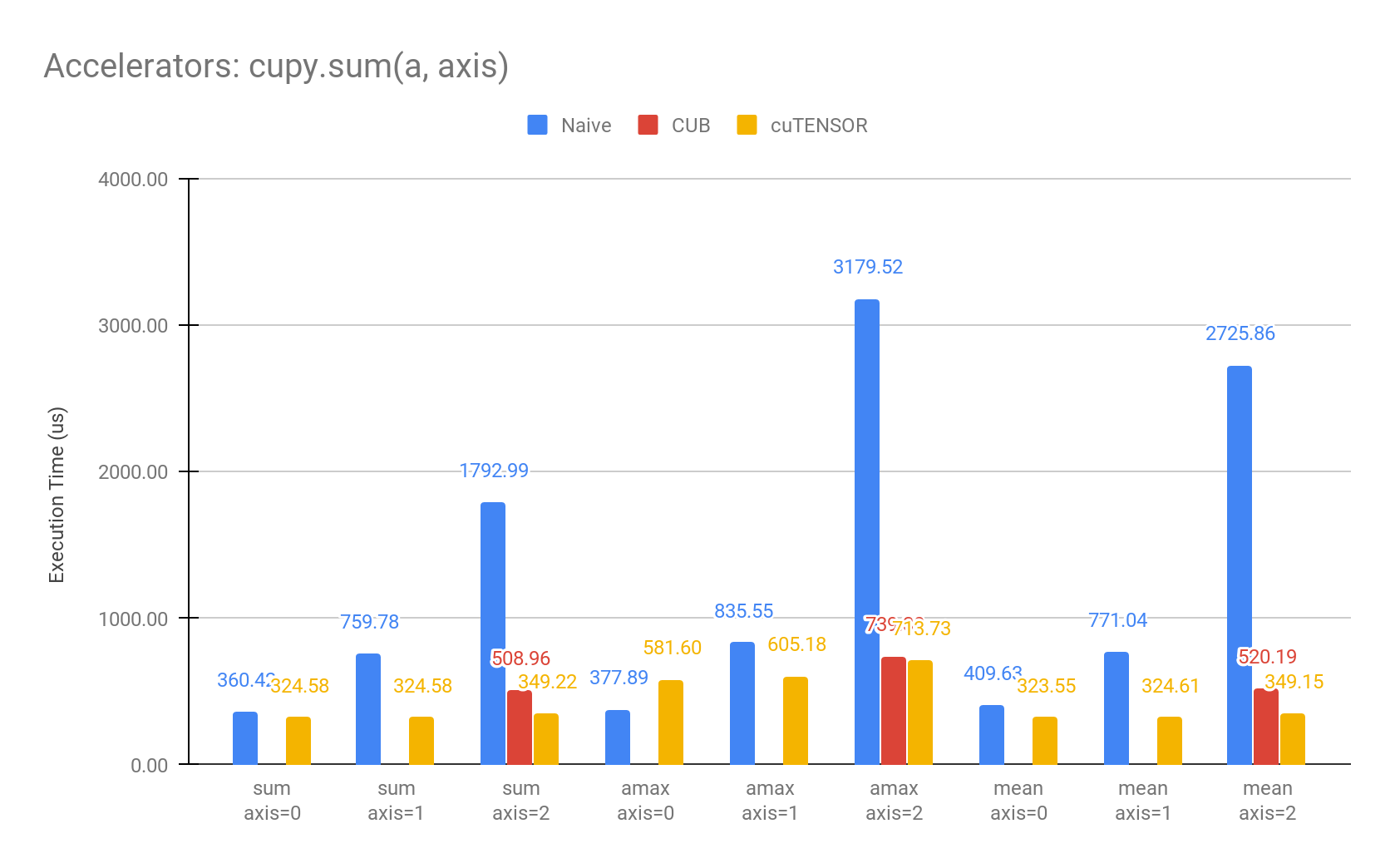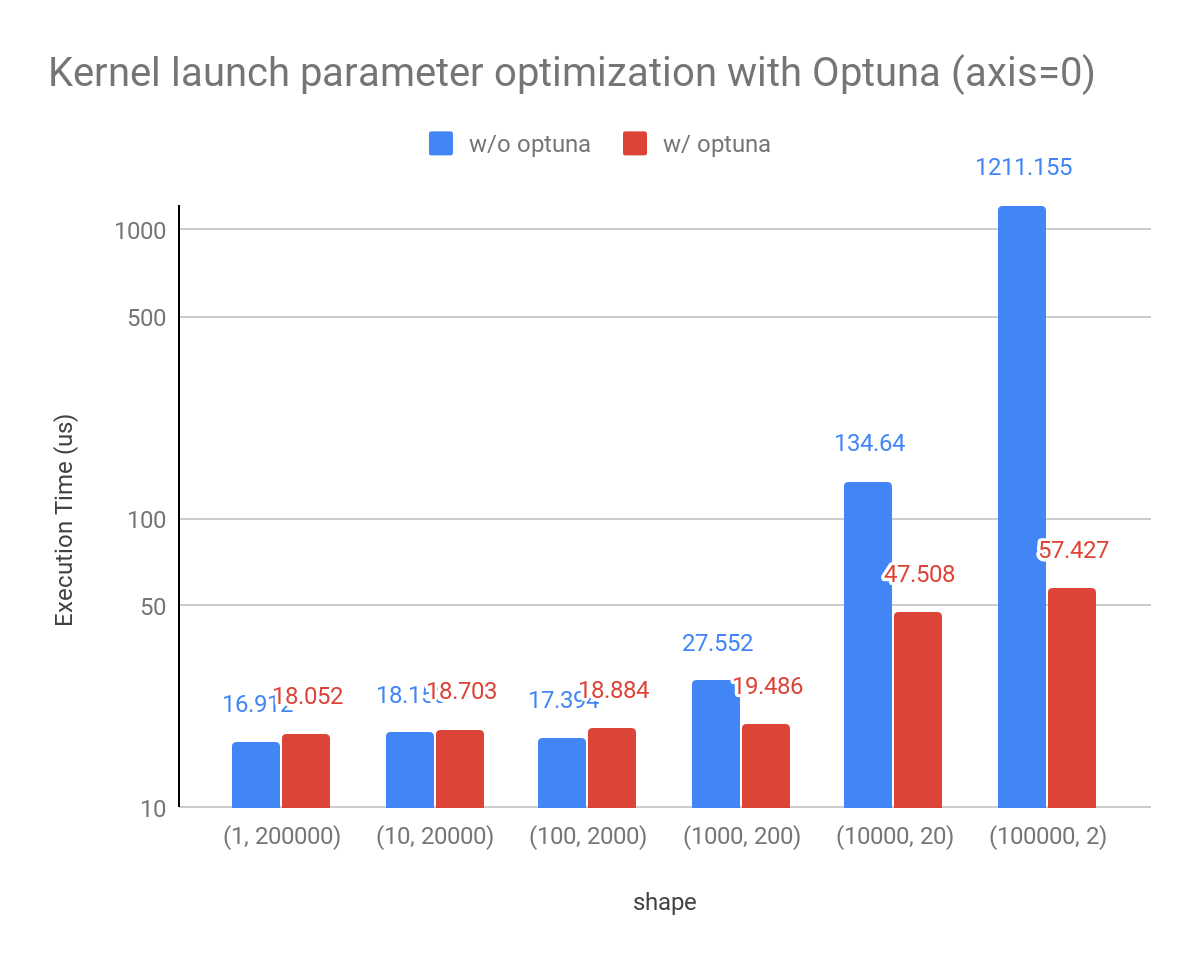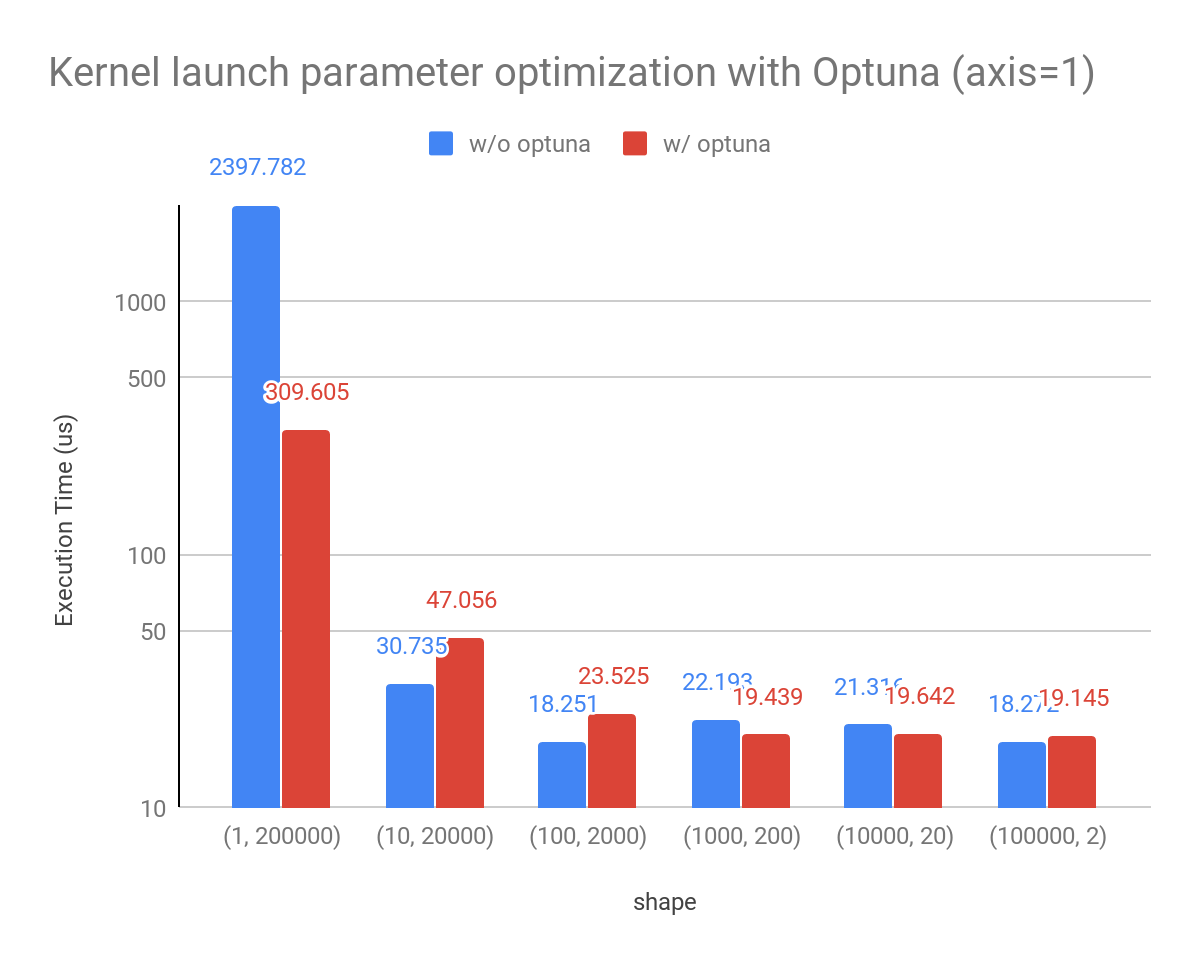Blog

Today, CuPy v8.0.0 has been released to the public. The most relevant features of this version are as follows:

• Use of TF32 data type for matrix multiplication.
• Enable CUB and cuTENSOR to improve performance of several routines.
• Improved kernel fusion with support for interleaved reductions.
• Use of Optuna to automatically tune kernel launching parameters to improve performance.
• Memory pool interoperability with PyTorch and other frameworks.

To see the list of all the improvements and bug corrections please refer to the release note.

## Performance Improvement Using TF32

With the new NVIDIA Ampere architecture and available starting CUDA 11, it is now possible to use TensorFloat-32 for matrix multiplications. In CuPy v8, we provide transparent support for it when using `float32` in routines such as `cupy.matmul`, `cupy.tensordot` resulting in noticeable performance improvements.Environment: NVIDIA Ampere A100, CUDA11

This feature is only available in GPUs with compute capability 8.0. To enable it, set the CUPY_TF32 environment variable as shown in the command line below.

`\$ CUPY_TF32=1 python run.py`

## Performance Improvement Using CUB and cuTENSOR

For several routines in CuPy, it is possible to use the CUB and cuTENSOR libraries to further improve performance. For example, we show the results achieved when using both libraries to accelerate the `cupy.sum` reduction operation.

For example, given a 1D array of 400000 float32  elements, the following graph shows the execution time improvement.Environment: NVIDIA Tesla V100, CUDA11

When applying the same operation to a multidimensional array of shape=(400, 400, 400), the following graph shows the results when the reduction is applied to different axes.Environment: NVIDIA Tesla V100, CUDA11

In the current version, CUB is only available when the axes in the reduction are contiguous in memory (the axis=2 case). We plan to address this in upcoming releases.

When using an external library to achieve performance improvements in CuPy, we have added an interface called accelerators. By default, accelerators is not set, but should you want to try CUB, and cuTENSOR for your operations, please set the CUPY_ACCELERATORS environment variable as follows:

`\$ CUPY_ACCELERATORS=cub,cutensor python run.py`

## Improved Kernel Fusion

While combining multiple kernels into a single one using `cupy.fuse`, it was only possible to use a single reduction operation (cupy.sum, etc.) at the end. With the new kernel fusion mechanism available in CuPy v8.0.0, now it is possible to combine multiple element wise operations with interleaved reductions as shown in the next code snippet.

```@cupy.fuse()
def batchnorm(x, gamma, beta, running_mean, running_var, size, adjust):
decay = 0.9
eps = 2e-5
expander = (None, slice(None), None, None)

gamma = gamma[expander]
beta = beta[expander]
mean = cupy.sum(x, axis=(0, 2, 3)) / size
diff = x - mean[expander]
var = cupy.sum(diff * diff, axis=(0, 2, 3)) / size
inv_std = 1. / cupy.sqrt(var + eps)
y = gamma * diff * inv_std[expander] + beta

running_mean *= decay
running_mean += (1 - decay) * mean
running_var *= decay
running_var += (1 - decay) * adjust * var
return y```

## Tuning Kernel Launch Parameters with Optuna

When doing reduction operations in CuPy, the kernels are very sensitive to the CUDA block size, and other parameters that are difficult to estimate. We use Optuna to automatically discover the best parameters to launch such kernels resulting in a greatly improved performance in several cases.

In previous versions, although there were heuristics to determine those parameters.  Depending on the GPU model and other variables it was not always possible to find a highly performant configuration. Now, CuPy implements a mechanism to find these parameters and cache them for future kernel invocations.

Given a `float32` array of 200000, we show the performance results below while changing its shape.Environment: NVIDIA Tesla V100, CUDA11

This feature is available by using cupyx.optimizing.optimize as shown in the next example

```import cupyx.optimizing

with cupyx.optimizing.optimize():
y = cupy.sum(x)```

## Sharing the Memory Pool with External Libraries

To use external libraries such as PyTorch memory pool in CuPy, the cupy.cuda.memory.PythonFunctionAllocator feature has been added. For example, the user can provided both, malloc_func, free_func functions to interface with an external memory pool as shown in the next snippet:

```python_alloc = cupy.cuda.memory.PythonFunctionAllocator(malloc_func, free_func)
cupy.cuda.memory.set_allocator(python_alloc.malloc)```

If you want to combine CuPy with PyTorch, we have simplified this process in pytorch-pfn-extras (v0.3.1).

By calling pytorch_pfn_extras.cuda.use_torch_mempool_in_cupy() CuPy will retrieve the GPU memory directly from the PyTorch memory pool, resulting in a more efficient memory utilization and reduced fragmentation when combining CuPy and PyTorch to preprocess data or use custom kernels in PyTorch functions. Please take a look at the  pytorch-pfn-extras documentation.